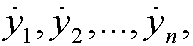6. The general case.

We define the n canonical momentumsfor the general action integralIf we assume thatwe can via the implicit function theorem solve the system of momentums for the variablesand getWe now define the Hamiltonian byBy using similar arguments as in the previous part we get Hamilton’s equations:This is a system of 2n first order ordinary differential equations in the unknown funktions y1,y2,…,yn, and the unknown momentums p1,p2,…,pn. The system contains the same information as the corresponding n second order Euler-Lagrange’s equations